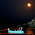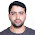Friday, September 7, 2012

MATLAB - 1D Schrodinger wave equation (Time independent system)

schrodinger
% =========================================================================
%                Program to solve 1D infinite well problem
% ***************************** By Mahesha MG *****************************
% This is to solve 1D Schrodinger wave equation (Time independent system)
% by finite difference method -- boundary value problem

% Date: 07/09/2012
% =========================================================================
clc;
clear;
x0=0;
m=1.1;
sum2=1;
n=input('Enter energy state n: ');
xl=input('Enter length of the well(in nm): ');
while(floor(m)<m)
h=input('Enter stepsize in nm: ');
m=(xl-x0)/h;
if floor(m)<m
warning('Step size is wrong. Enter proper value')
end

end
x=x0:h:xl;
y=zeros(size(x));
y(1)=0;
y(m+1)=0;
y(2)=1;
for i=3:m
y(i) = -(y(i-2)+((-2+(n*n*pi*pi*h*h/(xl*xl)))*y(i-1)));
sum2=sum2+y(i)^2;
end
y=y/sqrt(sum2); %normalization
y2=y.^2;       %y2 gives probability density
figure('color','white')
subplot(2,1,1), plot(x,y,'r')
title('By Mahesha MG')
xlabel('Distance in nm')
ylabel('wave function')
subplot(2,1,2),plot(x,y2)
xlabel('Distance in nm')
ylabel('probability density')

1.MMG IS A GREAT Physicist.

2.3.can i have a matlab code for 1D wave equation or even 2D please..i am stuck with an assignment..thank you

4.is it possible to have a solution without giving energy eigen values . i mean by diagonalizing the hamiltonian.

5.i need code MATLAB - 1D Schrodinger wave equation (Time dependent system) for a harmonic oscillator ,plzzzzzz

6.pls tell me how to code S.E of a particle constrain in a uniform magnetic field using fourth order runge-kutta?

7.how to write code for lowest even solution of schrodinger equation

8.Which metod it is executed with

9.finite diff method